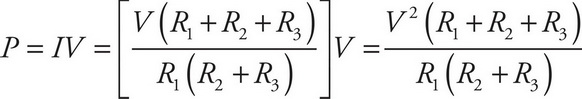# AP Physics 1 Question 366: Answer and Explanation

### Test Information

Question: 366

4. Question refers to the following figure: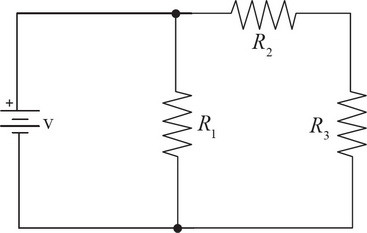Determine the total power dissipated through the circuit shown above in terms of V, R1, R2, and R3.

• A.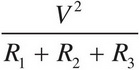• B.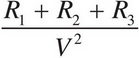• C.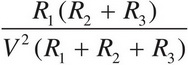• D.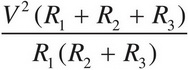Explanation:

D

First, replace all the resistors with an equivalent resistor.

R2 and R3 are in series, so R2 and 3, = R2 + R3.

R1 and R2 and 3 are in parallel, so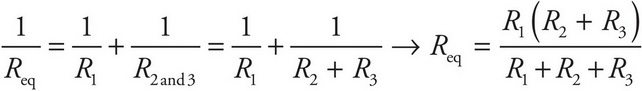.

Second, calculate the current coming out of the battery,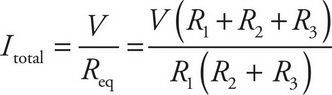Finally, calculate the power,